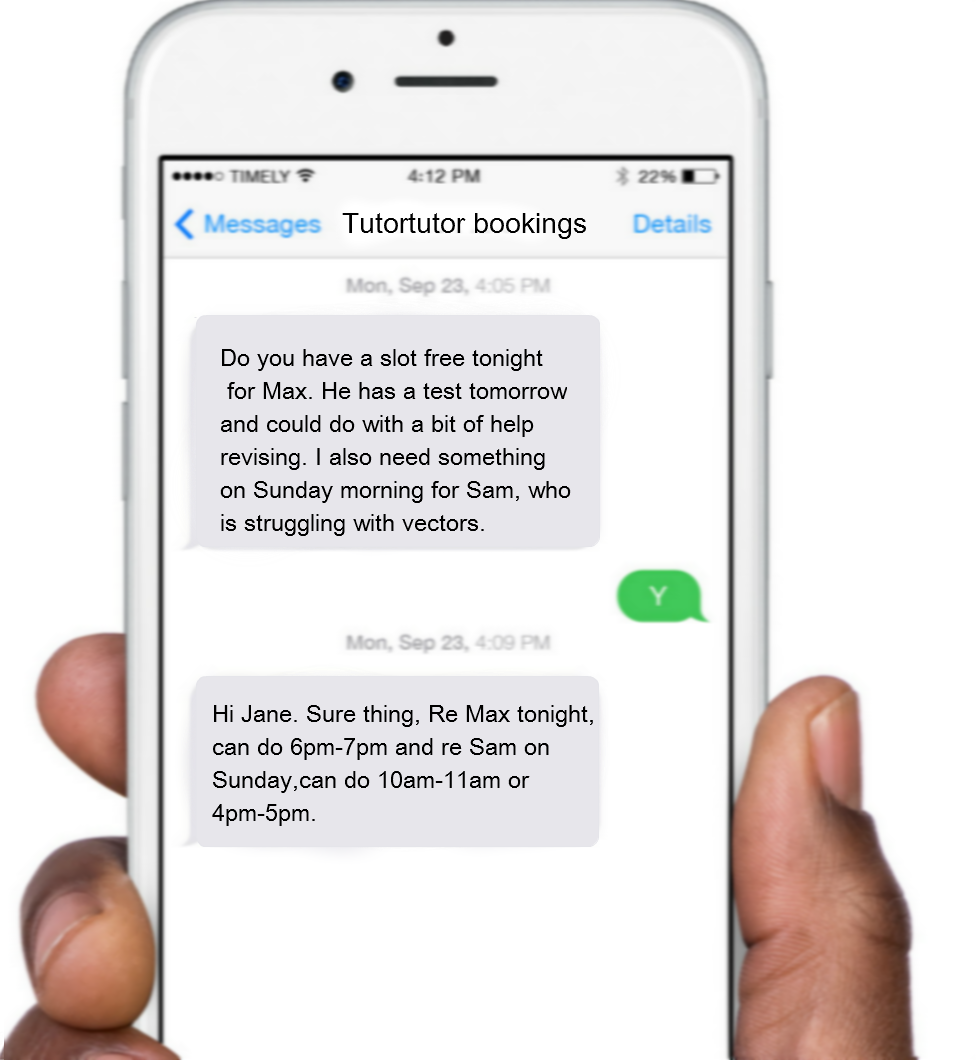Booking is very flexible and personal at Tutortutor. Simply send us your requirements and let us make the necessary arrangements for you. We are also one of the few online tutoring agencies where you can book lessons at short notice, up to 30mins before each scheduled lesson, provided that your tutor is available.

.

#### HNC 1-2-1 Tuition

Struggling with questions like these. Get One to One tuition with our trained HNC Engineering Tutors Q2 A beam of rectangular cross section 200 mm deep and 100 mm wide. If the beam is 3m long, simply supported at either end and carries point loads as shown in FIGURE 2 (a) Calculate the maximum bending moment (b) Calculate the maximum stress in the beam   3. A mass of 0.5 kg is suspended from a flywheel as shown in FIGURE 2. If the mass is released from rest and falls a distance of 0.5 m in 1.5 s, calculate: (a) The linear acceleration of the mass. (b) The angular acceleration of the wheel.   Help is Just One Click Away